# Wave Propagation in Step-Index Fibers

This tutorial considers propagation of light in step-index fibers by using Maxwell's equations for electromagnetic waves. We will discuss the concept of fiber modes where fiber is shown to support a finite number of guided modes. We also consider how a step-index fiber can be designed to support only a single mode and the properties of single-mode fibers.

#### 1. Maxwell's Equations

Like all electromagnetic phenomena, propagation of optical fields in fibers is governed by Maxwell's equations. For a nonconducting medium without free charges, these equations take the form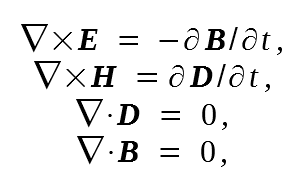where E and H are the electric and magnetic field vectors, respectively, while D and B are the corresponding electric and magnetic flux densities. The flux densities are related to the field vectors by the constitutive relations given bywhere ε0 is the vacuum permittivity, μ0 is the vacuum permeability, and P and M are the induced electric and magnetic polarizations, respectively. For optical fibers M = 0 because of the nonmagnetic nature of silica glass.

Evaluation of the electric polarization P requires a microscopic quantum-mechanical approach. Although such an approach is necessary when the optical frequency is near a medium resonance, a phenomenological relation between P and E can be used far from medium resonances. This is the case for optical fibers in the wavelength region 0.5~2 μm, a range that covers the low-loss region of optical fibers that is of interest for fiber-optic communication systems. In general, the relation between P and E can be nonlinear. Although the nonlinear effects in optical fibers are of considerable interest and will be covered in other tutorials, they can ignored in the discussion of fiber modes. P is then related to E by the relation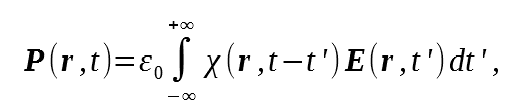where the linear susceptibility χ is, in general, a second-rank tensor; it reduces to a scalar for an isotropic medium. Since silica glass is an isotropic material, we assume χ to be scalar. Optical fibers become slightly birefringent when isotropy is destroyed by intentional or unintentional variations in the core shape or by a nonuniform stress; such birefringent effects are considered in a separate tutorial. The above equation assumes a spatially local response. However, it includes the nonlocal nature of the temporal response, a feature that has important implications for optical fiber communications through chromatic dispersion.

The previous equations provide a general formalism for studying wave propagation in optical fibers. In practice, it is convenient to use a single field variable E. By taking the curl of the first equation and using the others, we obtain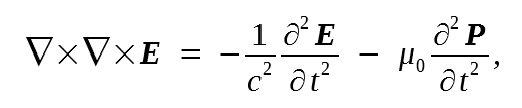where the velocity of light c is defined as c = (μ0ε0)-1/2. By introducing the Fourier transform of E(r,t) through the relation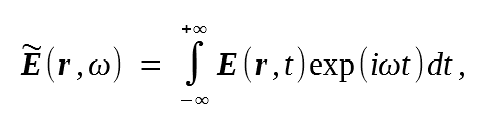as well as a similar relation for P(r,t), and by using other equations, we get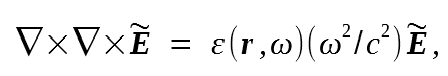where the frequency-dependent dielectric constant is defined asandis the Fourier transform of χ(r, t). In general, ε(rω) is complex. Its real and imaginary parts are related to the refractive index n and the absorption coefficient α by the definition

ε = (n + iαc/2ω)2

By using the last two equations n and α are related to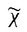as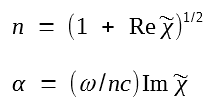where Re and Im stand for the real and imaginary parts, respectively. Both n and α are frequency dependent. The frequency dependence of n is referred to as chromatic dispersion or simply as material dispersion. In the next section, it is shown to limit the performance of fiber-optic communication systems in a fundamental way.

Two further simplifications can be made. First, ε can be taken to be real and replaced by n2 because of low optical losses in silica fibers. Second, since n(rω) is independent of the spatial coordinate r in both the core and the cladding of a step-index fiber, one can use the identity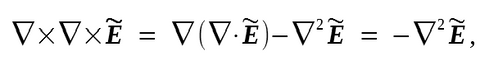where we used the relation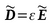to set. This simplification is made even for graded-index fibers.

The above equation then holds approximately as long as the index changes occur over a length scale much longer than the wavelength. We then obtainwhere the free-space wave number is defined as

k0ω/c = 2π/λ

and λ is the vacuum wavelength of the optical field oscillating at the frequency ω. This equation will be solved to obtain the optical modes of step-index fibers.

#### 2. Fiber Modes

The concept of the mode is a general concept in optics occurring, for example, int he theory of lasers. An optical mode refers to a specific solution of the wave equation that satisfies the appropriate boundary conditions, and has the property that its spatial distribution does not change with propagation.

The fiber modes can be classified as guided modes, leaky modes, and radiation modes. As one might expect, signal transmission in fiber-optic communication systems takes place through the guided modes only. The following discussion focuses exclusively on the guided modes of an ideal fiber with an infinitely thick cladding layer.

To take advantage of the cylindrical symmetry, we rewrite the wave equation in the cylindrical coordinates ρ, Φ, and z aswhere for a step-index fiber of core radius a, the refractive index n is of the form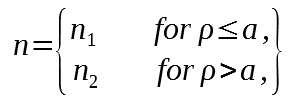For simplicity of notation, the tilde overhas been dropped and the frequency dependence of all variables is implicitly understood. The above wave equation is written for the axial component Ez of the electric field vector. Similar equations can be written for the other five components of E and H. However, it is not necessary to solve all six equations, since only two components out of six are independent. It is customary to choose Ez and Hz as the independent components and obtain Eρ, EΦ, Hρ, and HΦ in terms of them.

The wave equation can be solved by using the method of separation of variables and writing Ez as

Ez(ρ,φ,z) = F(ρ)Φ(φ)Z(z)

We then obtain three ordinary differential equations: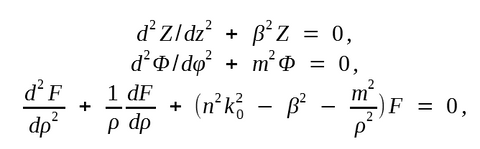We also get a solution of the form Z = exp(iβz), where β has the physical significance of the propagation constant. Similarly there is a general solution Φ = exp(imφ); the constant m is restricted to take only integer values, since the field must be periodic in φ with a period of 2π.

The last equation is a well-known differential equation for the Bessel functions. Its general solution in the core and cladding regions is given by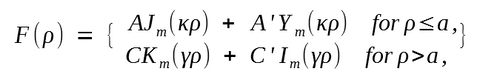where A, A', C, and C' are constants and Jm, Ym, Km, and Im are different kinds of Bessel functions. The parameters κ and γ are defined by:

κ2 = n12k02 - β2
γ2 = β2 - n22k02

Considerable simplification occurs when we use the boundary condition that the optical field for a guided mode should be finite at ρ = 0 and decay to zero at ρ = ∞. Since Ym(κρ) has a singularity at ρ = 0, F(0) can remain finite only if A' = 0. Similarly, F(ρ) vanishes at infinity only if C' = 0. The general solution is thus of the form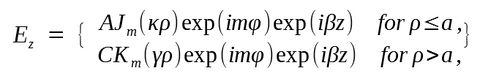The same method can be used to obtain Hz. Indeed, the solution is the same but with different constants B and D, that isThe other four components, Eρ, Eφ, Hρ, and Hφ, can be expressed in terms of Hz and Ez by using Maxwell's equations. The result is:The above equations express the electromagnetic field in the core and cladding regions of an optical fiber in terms of four constants, A, B, C, and D. These constants are determined by applying the boundary condition that the tangential components of E and H be continuous across the core-cladding interface. By requiring the continuity of Ez, Hz, Eφ, and Hφ at ρ = a, we obtain a set of four homogeneous equations satisfied by A, B, C, and D. These equations have a nontrivial solution only if the determinant of the coefficient matrix vanishes. This condition provides us with an eigenvalue equation whose solutions determine the propagation constant β. Since the algebraic details are straightforward, we write the eigenvalue equation directly: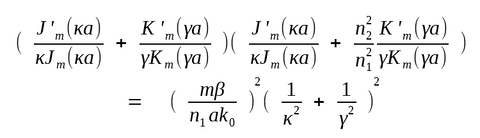where a prime indicates differentiation with respect to the argument.

For a given set of the parameters k0, a, n1, and n2, the eigenvalue equation can be solved numerically to determine the propagation constant β. In general, it may have multiple solutions for each integer value of m. It is customary to enumerate these solutions in decreasing numerical order and denote them by βmn for a given m (n = 1, 2, ...).

Each value βmn corresponds to one possible mode of propagation of the optical field whose spatial distribution is obtained from above equations. Since the field distribution does not change with propagation except for a phase factor and satisfies all boundary conditions, it is an optical mode of the fiber.

In general, both Ez and Hz are nonzero (except for m = 0), in contrast with the planar waveguides, for which one of them can be taken to be zero. Fiber modes are therefore referred to as hybrid modes and are denoted by HEmn or EHmn depending on whether Hz > Ez or vice versa.

In the special case of m = 0, HE0n and EH0n are also denoted by TE0n and TM0n, respectively, since they correspond to either transverse-electric (Ez = 0) or transverse-magnetic (Hz = 0) modes of propagation. A different notation LPmn is also used for weakly guiding fibers for which both Ez and Hz are nearly zero (LP stands for linearly polarized modes).

A mode is uniquely identified by its propagation constant β. It is useful to introduce a quantity; it is called the mode index (or effective index) and has the physical significance that the mode propagates with an effective refractive index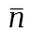whose value lies in the range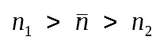. A mode ceases to be guided when. This can be understood by noting that the optical field of guided modes decays exponentially inside the cladding layer, sinceWhen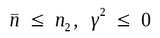, and the exponential decay does not occur. The mode is said to reach cutoff when γ becomes zero or when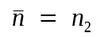. From previous equations,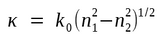when γ = 0. A parameter that plays an important role in determining the cutoff condition is defined as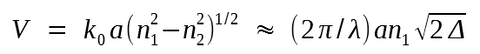It is called the normalized frequency (V ∝ ω) or simply the V parameter. It is also useful to introduce a normalized propagation constant b defined by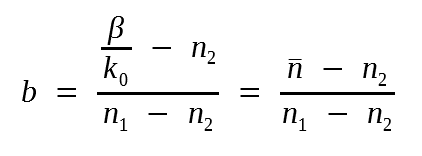The following figure shows a plot of b as a function of V for a few low-order fiber modes obtained by solving the eigenvalue equation.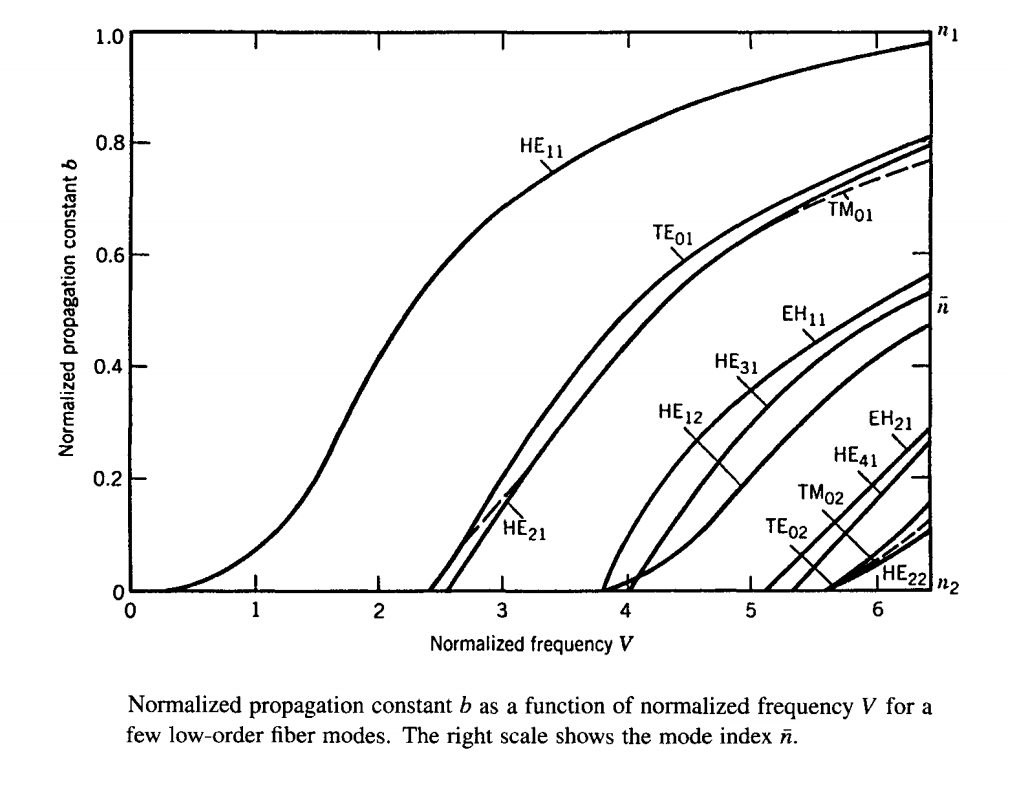A fiber with a large value of V supports many modes. A rough estimate of the number of modes for such a multimode fiber is given by V2/2. For example, a typical multimode fiber with a = 25 μm and Δ = 5 x 10-3 has V ≈ 18 at λ = 1.3 μm and would support about 162 modes. However, the number of modes decreases rapidly as V is reduced. As seen in the figure, a fiber with V = 5 supports seven modes. Below a certain value of V all modes except the HE11 mode reach cutoff. Such fibers support a single mode and are called single-mode fibers.

#### 3. Single-Mode Fibers

Single-mode fibers support only the HE11 mode, which is also known as the fundamental mode of the fiber. The fiber is designed in such a way that all higher-order modes are below cutoff at the operating wavelength. As seen in the figure above, the V parameter determines the number of modes supported by a fiber. The cutoff condition of various modes is also determined by V. The fundamental mode has no cutoff and is always supported by a fiber.

1) Single-Mode Condition

The single-mode condition is determined by the value of V at which the TE01 and TM01 modes reach cutoff. The eigenvalue equation for these two modes can be obtained by setting m = 0 and is given by:A mode reaches cutoff when γ = 0. Since κa = V when γ = 0, the cutoff condition for both modes is simply given by:

J0(V) = 0

The smallest value of V for which J0(V) = 0 is 2.405. A fiber designed such that V < 2.405 supports only the fundamental HE11 mode. This is the single-mode condition.

We can estimate the core radius of single-mode fibers used in lightwave systems. For the operating wavelength range 1.3-1.6 μm, the fiber is generally designed to become single mode for λ > 1.2 μm. By taking λ = 1.2 μm, n1 = 1.45, and Δ = 5 x 10-3, we get that V < 2.405 for a core radius a < 3.2 μm. The required core radius can be increased to about 4 μm by decreasing Δ to 3 x 10-3. Indeed, most telecommunication fibers are designed with a ≈ 4 μm. The core radius is smaller by about a factor of 2 for fibers designed to operate in a single mode in the visible region of the optical spectrum.

2) Mode Index

The mode indexat the operating wavelength can be obtained according to:and by using the b versus V figure above, which provides b as a function of V for the HE11 mode.

An analytic approximation for b is:

b(V) ≈ (1.1428 - 0.9960/V)2

and is accurate to within 0.2% for V in the range 1.5-2.5.

3) Field Distribution

The field distribution of the fundamental mode is obtained by using equations above. The axial components Ez and Hz are quite small for Δ << 1. Thus the HE11 mode is approximately linearly polarized for weakly guiding fibers. It is also denoted as LP01, following an alternative terminology in which all fibers modes are assumed to be linearly polarized.

One of the transverse components can be taken as zero for a linearly polarized mode. If we set Ey = 0, the Ex component of the electric field for HE11 mode is given by: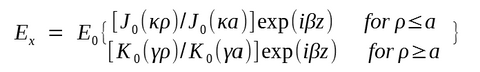where E0 is a constant related to the power carried by the mode. The dominant component of the corresponding magnetic field is given by

Hy = n200)1/2Ex

Such a mode is linearly polarized along the x axis. The fiber also supports another mode linearly polarized along the y axis. In this sense a single-mode fiber actually supports two orthogonally polarized modes that are degenerate, as they have the same mode index.

4) Birefringence

The degenerate nature of the orthogonally polarized modes holds only for an ideal single-mode fiber with a perfectly cylindrical core of uniform diameter. Real fibers exhibit considerable variation in the shape of their core along the fiber length. They may also experience nonuniform stress such that the cylindrical symmetry of the fiber is broken. Degeneracy between the orthogonally polarized fiber modes is removed because of these factors, and the fiber acquires birefringence. The degree of birefringence is defined bywhereand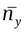are the mode indices for the orthogonally polarized fiber modes.

Birefringence leads to a periodic power exchange between the two polarization components. The period, referred to as the beat length, is given by

LB = λ/B

Typically, B ~ 10-7, and LB ~ 10 m for λ ~ 1 μm.

From a physical viewpoint, linearly polarized light remains linearly polarized only when it is polarized along one of the principle axes. Otherwise, its state of polarization changes along the fiber length from linear to elliptical, and then back to linear, in a periodic manner over the length LB.

The following figure shows schematically such a periodic change in the state of polarization for a fiber of constant birefringence B. The fast axis in this figure corresponds to the axis along which the mode index is smaller. The other axis is called the slow axis.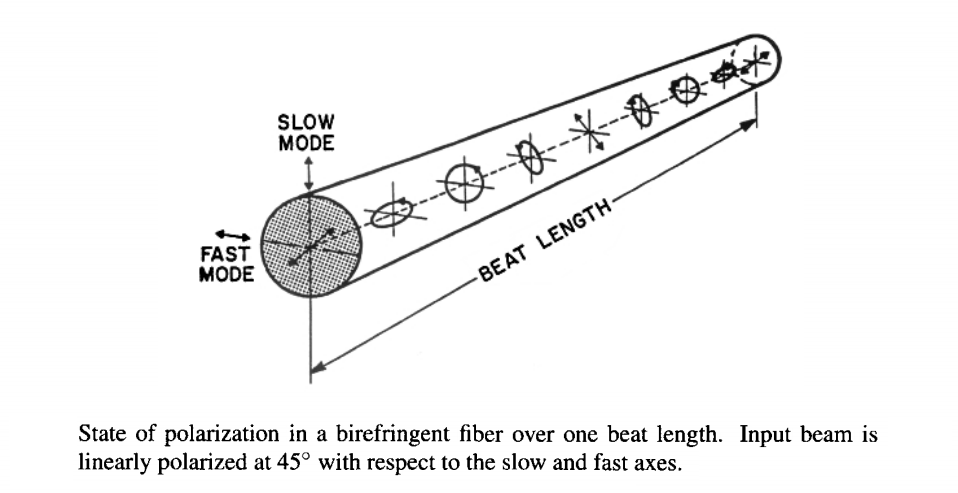In conventional single-mode fibers, B is not constant along the fiber but changes randomly because of fluctuations in the core shape and the nonuniform stress acting on the core. As a result, light launched into the fiber with linear polarization quickly reaches a state of arbitrary polarization. The unknown polarization state is generally not a problem for optical communication systems whose receives detect the total intensity directly. However, it becomes of concern for coherent communication systems for which it becomes necessary to employ polarization-insensitive coherent receivers. It is possible to make fibers for which random fluctuations in the core shape and size are not the governing factor in determining the state of polarization. Such fibers are called polarization-preserving fibers. A large amount of birefringence is introduced intentionally in these fibers through design modifications so that small random birefringence fluctuations do not affect the light polarization significantly. Typically, B ~ 10-4 for such fibers.

5. Spot Size

Since the field distribution given by previous equations is cumbersome to use in practice, it is often approximated by a Gaussian distribution of the form

Ex = A exp(-ρ2/w2) exp(iβz)

where w is the field radius and is referred to as the spot size. It is determined by fitting the exact distribution to the Gaussian function or by following a variational procedure. The following figure shows the dependence of w/a on the V parameter. A comparison of the actual field distribution with the fitted Gaussian is also shown for V = 2.4. The quality of fit is generally quite good for the values of V in the neighborhood of 2.The spot size w can be determined from the last figure. It can also be determined from an analytic approximation accurate to within 1% for 1.2 < V < 2.4 and given by

w/a ≈ 0.65 + 1.619-3/2 + 2.879-6

The power contained in the core is given byThe last two equations determine the fraction of the mode power contained inside the core for a given value of V. Although nearly 75% of the mode power resides in the core for V = 2, this percentage drops down to 20% for V ≈ 1. For this reason most telecommunication single-mode fibers are designed to operate in the range 2 < V < 2.4.